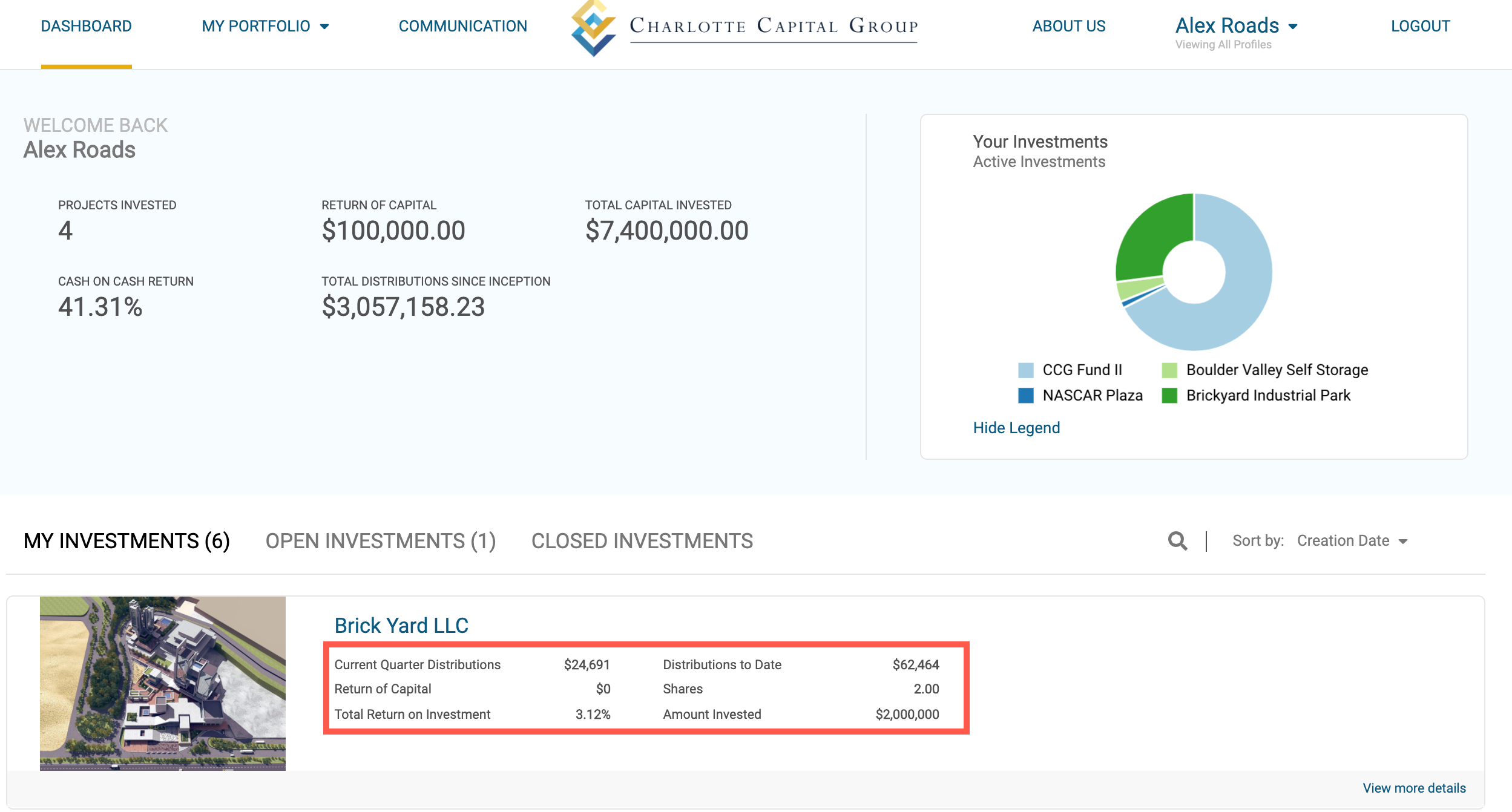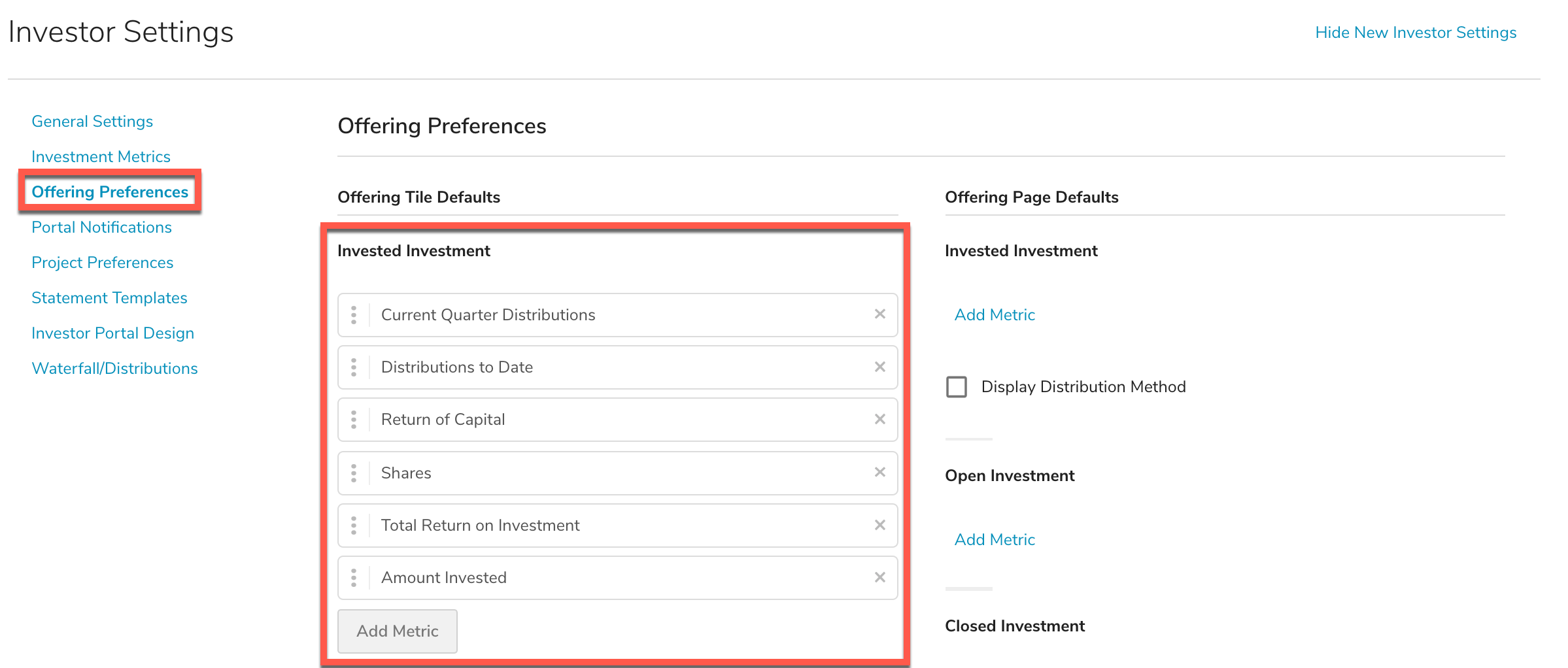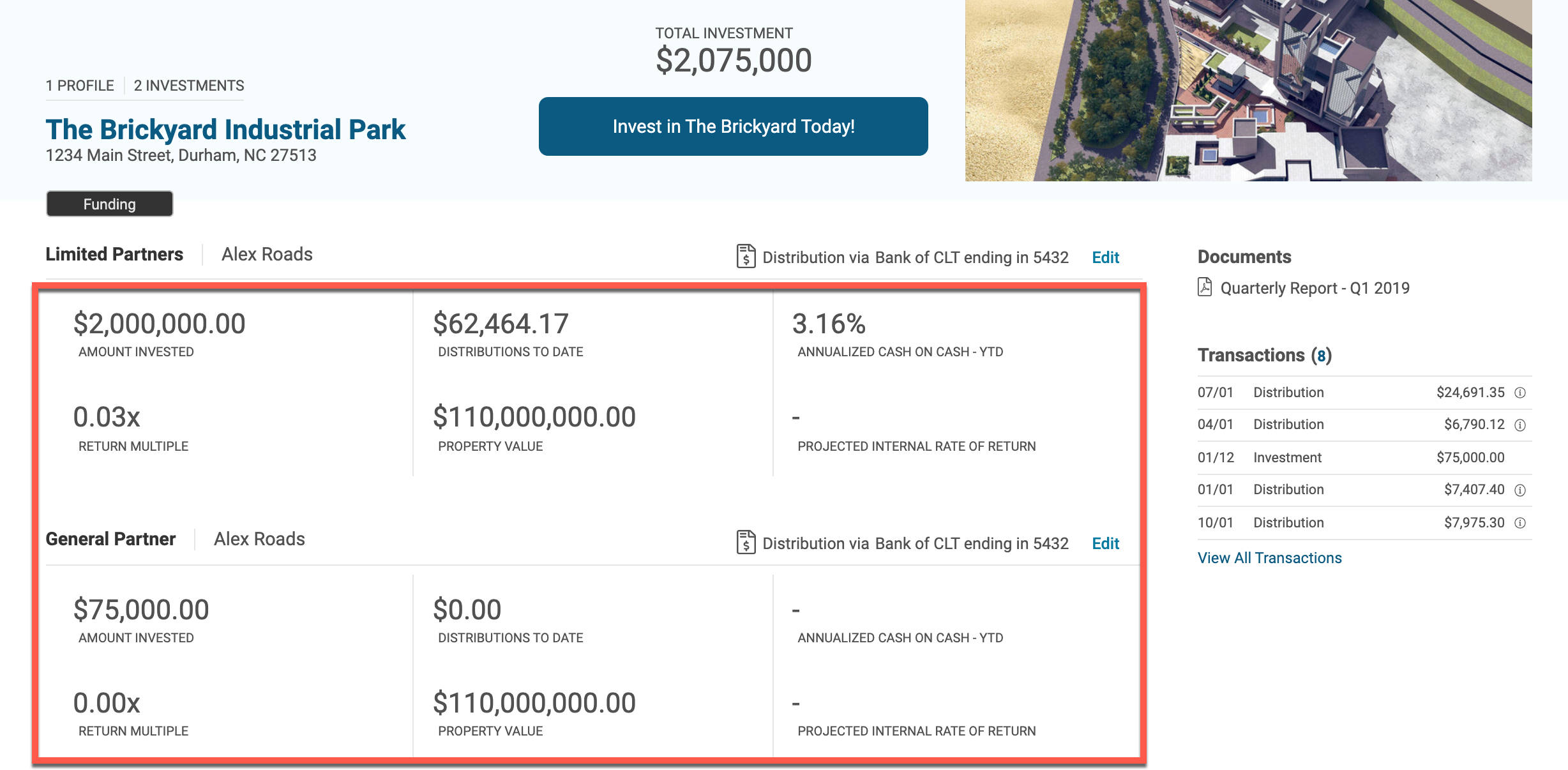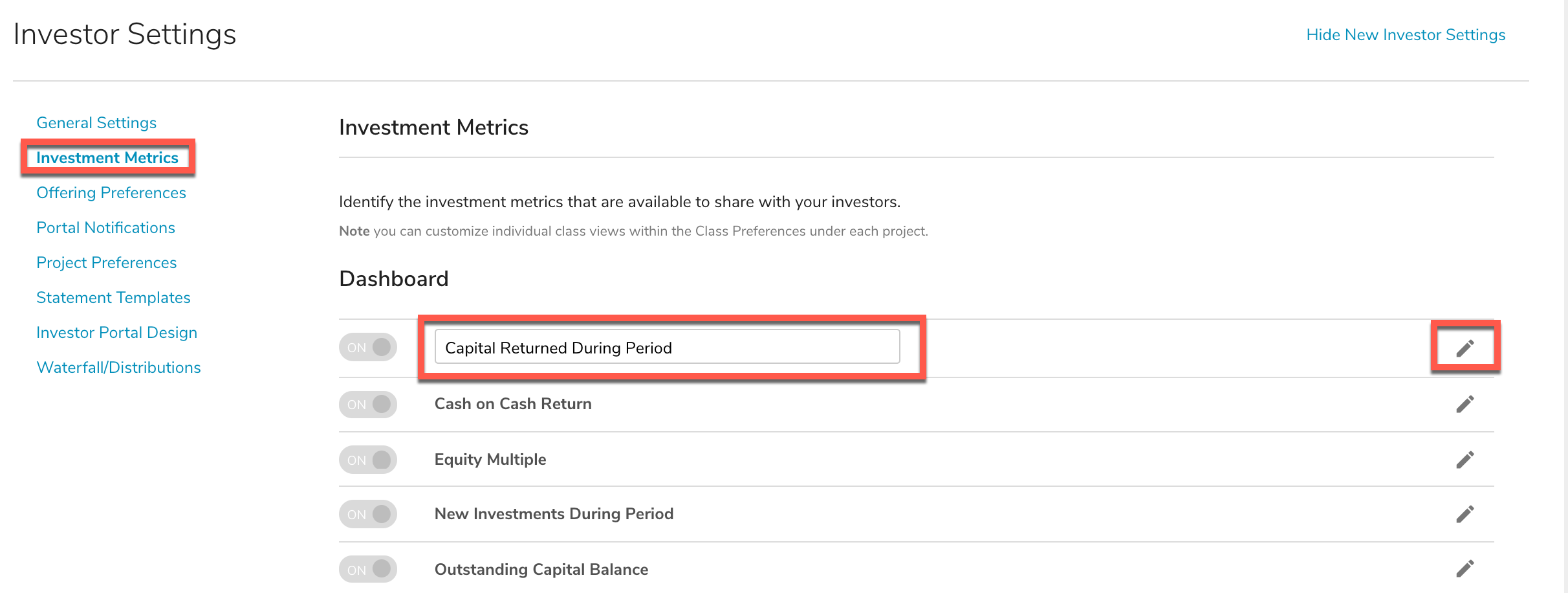# Offering Preferences (New Investor Portal)

Sponsors can pick and choose which Investment Metrics will be shown on the Investor Portal under My Investments, Open Investments and Closed Investments.To choose which investment metrics to display, go to Investor Settings > View New Investor Settings > Offering Preferences. These metrics are configured at the project level, not per individual class. You can select up to 6 metrics per status (Invested, Open, or Closed).Offering Tile Defaults are what displays on Investor Dashboards (as pictured in the image at the beginning of the article). Offering Page Defaults will display on the expanded offering page when an investor selects 'View More Details'. If the investor has placed investments in multiple classes, they will get information on both classes. Note - it is a good idea to choose universal metrics that apply to the majority of your deals. If you choose to display a metric and no information is available for that class, the metric will display with a -- through it, as pictured below.To rename any metrics, go to Investment Metrics and select the pencil icon next to the metric.Below is a list of Investment Metrics available and the associated calculations for each metric where applicable.

## Accrued and Unpaid Preferred Returns

All status’ – This is a calculated field based on your selected preferred return waterfall template. *Note the dashboard preference on period for preferred return.

Displays the accrued and unpaid preferred returns based on the specified dashboard preference date.## Acquisition Price

All status’ – This is a numerical field from the asset details page.
Displays the price paid to acquire the asset.

## Allocated Net Asset Value

Represents the Fund Net Asset Value or Property Net Asset Value as applicable times an Investor's ownership % in the Fund.

## Amount Invested

All status’ – This field will show the Amount Invested by the investment entity.

## Annualized Cash on Cash

Funded – This field will show the annualized cash income earned on the cash invested. Investment must have investment amount and at least one distribution with a value for the investment.

Liquidated - This field will show the annualized cash income earned on the cash invested. Investment must have investment amount and at least one distribution with a value for the investment, and an Exit Date for the project.

Users can select the following end date opens:

Distribution Period End Date

Distribution Period Pay Date

Prior Quarter End Date

Prior Month End Date

Prior Business Day (excluding Saturday and Sunday)

Inception to Date

Result = ((a)/(i)) x (365/L) x 100

1. A = sum of all distributions within the specified period
2. I = Investment Amount
3. L = DE-DS (+1)

a. DE = user selected end date

b. DS = Investment date

c. (+1) = system will add 1 day if the DE or DS value falls on the first or last day of the month

EXAMPLE:

DS = 01/25/2017

DE = 03/31/2019

L = 796

A = 87,500

I = 100,000

Result = ((87,500)/(100,000)) x (365/796) x 100 = 40.12%

Year to Date

Result = ((a)/(i)) x (365/L) x 100

1. A = sum of all distributions within the specified period
2. I = Investment Amount
3. L = DE-DS + 1

a. DE = user selected end date

b. DS = based on DE selection, 01/01/yyyy

EXAMPLE:

DS = 01/01/2019

DE = 03/31/2019

L = 90

A = 10,000

I = 100,000

Result = ((10,000)/(100,000)) x (365/90) x 100 = 40.56%

Trailing 3 Months

Result = ((a)/(i)) x (365/L) x 100

1. A = sum of all distributions within the specified period
2. I = Investment Amount
3. L = DE-DS (+1)

a. DE = user selected end date

b. DS = based on DE selection, 3 month look back

c. (+1) = system will add 1 day if the DE or DS value falls on the first or last day of the month

EXAMPLE:

DS = 01/01/2019

DE = 03/31/2019

L = 90

A = 10,000

I = 100,000

Result = ((10,000)/(100,000)) x (365/90) x 100 = 40.56%

Trailing 12 Month

Result = ((a)/(i)) x (365/L) x 100

1. A = sum of all distributions within the specified period
2. I = Investment Amount
3. L = DE-DS

a. DE = user selected end date

b. DS = based on DE selection, 365 day look back

EXAMPLE:

DS = 03/31/2018

DE = 03/31/2019

L = 365

A = 47,500

I = 100,000

Result = ((47,500)/(100,000)) x (365/365) x 100 = 47.5%

## Annualized Rate of Return

Funded – This is a calculated field.

### Calculation:

ds = Based on today's date, the start date of the period that the last distribution was distributed de = Based on today's date, the end date of the period that the last distribution was distributed a = Sum of all distributions within the specified period

b = Sum of "Return of Capital" distributions within the specified period c = Sum of all "Return of Capital" distributions

i = Investment amount

L = # of days in the specified period L = de – ds + 1

Result = ((a-b)/(i-c))*(365/L)*100

Investment must have invested amount, as well as at least one "Return of Capital" type distribution.

## Average Share Price

Displays the calculation of the (Sum of all Invested Capital)/(Sum of all Shares).

## Cash on Cash

All status’ – This is either a manual field or calculated based on the Type of Cash on Cash selected on the equity class. If manual, this field will display the Manual Cash on Cash Value entered. Will display with Custom Name for “Cash on Cash Return”, if entered. If calculated, Calculation: Cash on Cash = (Sum of Distributions / (Investment Amount) * 100. “Est.” will show when in Raising stage.

## (Est.) Closing Date

Raising –This field will show the estimated closing date of the project.

## Current Quarter Distributions

Funded – This field will display the sum of distributions made in the most current distribution period. Investment must have at least one distribution for the investment.

## Date Invested

Funded, Liquidated - This field will show the closing date for the project.

## Distributions to Date

Funded, Liquidated – Will show a sum of all distributions to date for the investment entity.

## Entity Ownership (%)

Funded, Liquidated – This field will show the Entity Ownership %. Investment amount and Project ownership structure definition must be complete for this field to display.

## Estimated Disposition Date

Raising, Funded – This is a numerical field from the asset details page.
Displays approximate date the asset is expected to be sold or liquidated.

## Fund Details

-Distributions
Shows the cumulative distributions paid to date by the investment.

-Expected Term
Displays the approximate term for the fund.

-Fund Hold Period
Shows the anticipated term for assets within the fund.

-Fund Image
Displays image uploaded to help identify a given fund.

-Net Asset Value
Displays the estimated net asset value of the fund.

-Target Amount
Displays the target amount you wish to raise for a given fund.

All status’ but only shows on fund projects. The inputs are found on the asset details page.

## Inception to Date Rate of Return

Funded – This is a calculated field.

### Calculation:

ds = Closing date of project de = Date of last distribution

a = Sum of all distributions within the specified period

b = Sum of "Return of Capital" distributions within the specified period c = Sum of all "Return of Capital" distributions

i = Investment amount

L = # of days in the specified period L = de – ds + 1

### Result = ((a-b)/(i-c))*(365/L)*100

Investment must have at least one “Return of Capital” type distribution. As well as a project close date defined on the equity class.## Estimated Internal Rate of Return

Displays the projected Internal Rate of Return for a project (entered manually on the Edit Classes page).

## Internal Rate of Return Calculation

Funded, Liquidated, Syndicating – This is a calculated field based on XIRR.
Displays the calculated internal rate of return for a project.

## Next Distribution Date

Funded – This field will show the next distribution date, given that a distribution frequency is defined on the equity class, and at least one distribution with a value is available for the investment.

## Preferred Return

Raising, Funded, Liquidated – This field will display the available preferred return if defined on the equity class.

## Projected Internal Rate of Return

Displays the projected Internal Rate of Return for each investors investment (calculated on the Projections tab).

## Property Value

All status’ – This displays the property value from the home page.
Displays the fair market value of a given piece of property.

## Rate of Return

Funded, Liquidated – While this will eventually be a calculated field, this field currently will display the Internal Rate of Return when defined on the equity class. We have scheduled development for a future date which will incorporate our client feedback, as Rate of Return is calculated differently across industries.

## Remaining Investment Term

Raising – This field will show the Project Close Date and Exit Date if defined in the project. Funded - This field will show the Project Exit Date if defined in the project.## Reporting for Period Ending ___

All status’ – This is a calculated field based off logic.
Informs investors that the metrics displayed are for the last period's distribution end date.

## Return Multiple

Funded, Liquidated – This is a calculated field.

Calculation:
(Sum of Distributions)/(Investment Amount)

*Investment must have invested amount, as well as at least one distribution.

## Return of Capital

Funded – This field displays a sum of all “Return of Capital” type distributions. Investment must have at least one “Return of Capital” type distribution for the investment.

## Shares

If issued, display a sum of all shares from all contributions.

## Total Return on Investment

Funded, Liquidated– This is a calculated field. Calculation: (Total Distributions – Return of Capital)/ Investment Amount.

## Unreturned Contributions

Funded – This is a calculated field. Calculation: (Investment amount – sum of “Return of Capital” type distributions).

## YTD Return of Capital

Funded – This field will show the year to date return of capital type distributions. Investment must have at least one Return of Capital type distribution for the investment.